top of page
Search

# Fractals: A Comprehensive Guide to Infinite Geometries!

Updated: Feb 14, 2021

Hi everybody! I'm back after winter break, and we're starting off 2020 on the right foot. We're looking at some of my favorite mathematical objects, fractals! Fractals are patterns that exist somewhere between the finite and infinite. As we'll see, they even have fractional dimensions (hence the name fractal) because they exist somewhere between integer dimensions! We'll look at how these seemingly impossible shapes exist when we allow ourselves to extend to infinity, in the third part of my infinity series (as promised)!

Before we start, let me define for you more clearly what a fractal is. A fractal is any shape that has infinite self-symmetry, meaning if you zoom in forever, you'll get a repeating pattern. Here's an example of what this zoom looks like: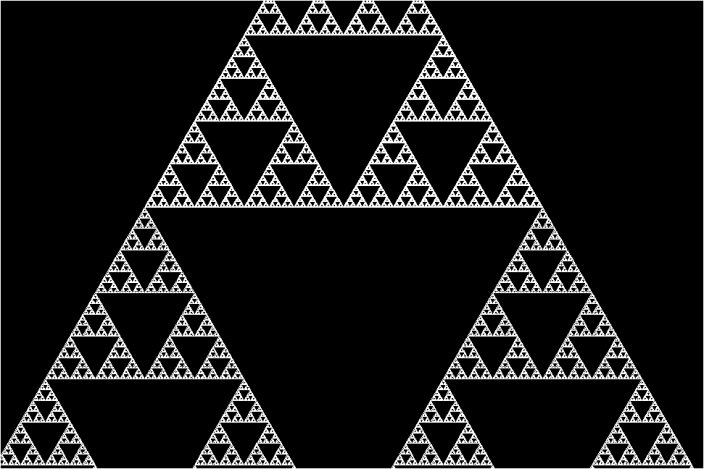Do you see what I mean by infinite self-symmetry? With each successive zoom, we see the same triangles! This infinite zoom is where infinity comes in! It turns out this makes for some pretty wacky properties, so let's look at some of my favorite fractals.

Koch's Snowflake

The first fractal we'll be looking at is Koch's Snowflake:It turns out that this snowflake has an infinite perimeter but a finite area!

Here's how we construct it. We start with an equilateral triangle and with each successive step, we "poke" out the middle third of each exposed side into another equilateral triangle. As we reach infinity, the shape is made up of an infinite number of line segments, so it becomes a curve!If you've had a bit of background with geometric series, I can explain to you why this shape has a finite area but an infinite perimeter. If you haven't, just skip on to the next section.

Let's look at the perimeter first. Let's say our equilateral triangle has sides of length 1.

Then, the perimeter of Step 1 is 3.

In Step 2, we're replacing each side of length 1 with four line segments of length 1/3, so each side is increasing by 1/3. Our total perimeter is then 3+3(1/3)=4.

In Step 3, we're replacing each side of length 1/3 with four line segments of length 1/9, so each side is increasing by 1/9. The number of sides has been multiplied by 4, so our total perimeter is then 4+12(1/9) = 4+(4/3)=16/3

In Step 4, we're replacing each side of length 1/9 with four line segments of length 1/27, so each side is increasing by 1/27. The number of sides has been multiplied by 4, so our total perimeter is then 16/3+48(1/27) = 16/3 + (16/9) = 64/9

Continuing this reasoning, in total, the perimeter is going to be the sum of the series 3+1+(4/3)+(16/9)+(64/27)+... which is a geometric series with ratio 4/3, which is greater than 1 (if you disregard the 3), so it's going to snowball to infinity.

Now let's look at the area. This time, let's assume our triangle has an area of 1.

In Step 1, the area is 1.

In Step 2, we're adding three triangles that have sides 1/3 of the original triangle, so their areas are 1/9 of the original triangle. Then, our total area is 1+3(1/9)=4/3

In Step 3, we're adding 12 triangles (remember the number of exposed sides are multiplied by 4 each time) that have areas 1/9 of the previous triangle or 1/9^2 = 1/81 of the original triangle. Then, our total area is 4/3 + 12/81 = 40/27

In Step 4, we're adding 48 (12*4) triangles that have areas 1/9 of the previous triangle or 1/9^3 = 1/729 of the original triangle. Then, our total area is 40/27+48/729 = 1128/729.

Continuing this reasoning, in total, the perimeter is going to be the sum of the series 1 + (3/9) + (12/81) + (48/729)+... which is a geometric series with ratio 4/9, which is less than 1, so it's going to converge on a value.

This value is going to be 1+ (1/3)/(1-(4/9)) = 1 + 9/15 = 8/5. (Here we use the a/(1-r) formula for infinite geometric series, so look it up if you don't know it!)

What all those calculations tell you is that the Koch snowflake is miraculous! It's almost impossible to consider a finite area and infinite perimeter, and yet, this snowflake embodies that. I absolutely love this fractal because it reveals some of the wonder of math, so yes, there's a reason it's my profile picture on here! :)

I want all my members to always be curious. Don't just accept the fact that this bizarre property is true for the Koch Snowflake; work it out! You'll learn more if you allow yourself to question and figure things out for yourself, and that's the reason I included these calculations here! We'll be looking at a couple other fractals that have similar crazy properties, and I advise you to work out why on your own! All you need is geometric series and a little bit of skill in setting it all up.

Before we move on, let me show you one other cool thing about the Koch snowflake: It can tessellate the plane! This means that if we place different sizes of snowflakes down, we can actually cover an entire surface with no gaps, which seems crazy for infinitely curved shapes. Here are two Koch patterns, but so many exist, so search up "koch snowflake tessellations" for more!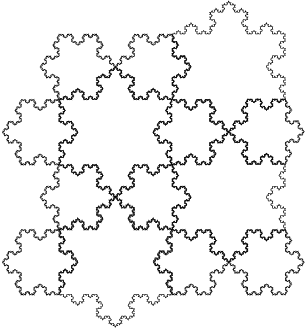Sierpinski Triangle

The second fractal we'll be looking at is the Sierpinski Triangle, a fractal you've seen both at the top of this article and in the GLeaM logo! Though I thought it best we start with the Koch snowflake, this one is actually my favorite because it shows up in such surprising places.Let's look at how this fractal is constructed.We begin with an equilateral triangle, and then we cut out the middle triangle of the overall triangle, and then we cut out the middles of those triangles, and then those... and you get the picture.

You can also create it by starting with your triangle and adding the successive triangles to the right and up from it, making it bigger and bigger instead of adding holes.

But, it turns out there are also some nonstandard ways of constructing the Sierpinski triangle! Here, we get the Sierpinski triangle from a square which we repeatedly reduce to 1/4 of its original on the top. It turns out you can use any other shape in a similar way too to get the Sierpinski triangle!Here, we construct the triangle not from a bunch of shapes but out of one continuous line, as we repeatedly add trapezoidal arcs! This is called the Sierpinski arrowhead.Here's one neat aspect of the Sierpinski triangle. It doesn't lie in a typical dimension. Here's how we'll think about this.

If you double a line in the first dimension, it becomes 2 times its original length.

If you double all the sides of a shape in the second dimension (think a square), it becomes 4 times its original area.

If you double all the sides of a solid in the third dimension (think a cube), it becomes 8 times its original volume.

What happens if you double the sides on a Sierpinski triangle? You get 3 times the original shape!

It seems that the number of times the object increases with doubling is 2 to the power of the dimension.

That means the dimension of the Sierpinski triangle is the number such that 2^x = 3. If you know what a logarithm is, the Sierpinski triangle's dimension is log_2(3) which is approximately 1.58496...

This is what we mean by a fractional dimension, a dimension that isn't an integer! (though to be fair, it isn't a fraction, so it's a bit of misnomer)

Let's look at a couple other interesting things related to the Sierpinski triangle!

If you've ever seen Pascal's triangle, it's a triangle where you begin with 1 on the top and sides of your triangle, and with each successive row, you add the two numbers above each cell to get that cell.

Pascal's triangle has an association with counting, such that if you pick the 2nd number (well, we start counting at zero, so really the 3rd number) in the 4th row (really 5th), it's the number of ways to pick 2 objects from a group of 4 objects. It's 6! Test it out.

The triangle has so many interesting properties, and I'd love to write an article on it in the future! Let me know in the comments below if that's something you'd like to see.

Anyway, it turns out that if you color all the odd numbers on Pascal's triangle black and all the even numbers white, you get the Sierpinski triangle! Think about why that's true because it's a really interesting problem!There are also many variations on the Sierpinski triangle, such as this one, so think about some other ways you can change it to make your own fractals!If I haven't blown your mind enough already, let me just say that the Sierpinski triangle pops up in many more places that I could possibly mention in this article from the field of chaos theory (where you essentially place points randomly according to a set of rules and somehow they create this triangle) to patterns that are generated from cells (sounds boring, but cellular automata are another amazing field I'd love to write an article on).

It's even related to the classic puzzle Towers of Hanoi, which I don't have space to explain, but it's a super fun game and puzzle that you can play by following that link, so check it out!

Normally, I wouldn't direct you to a Wikipedia article, but visit this one to see just how many relationships the Sierpinski triangle has with tons of visuals, or visit this other link by a UGA professor to learn more.

Sierpinski Carpet/Menger Sponge

We're going to be looking at a 3D fractal this time, but first we have to investigate the 2D version. This is called the Sierpinski Carpet:I think you can probably figure out how it's constructed, but here are the steps to creating it just in case: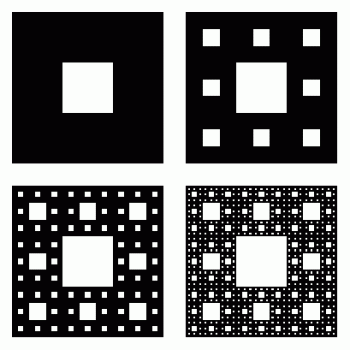Just like the triangle, we essentially cut out the middle square of all of our remaining squares in each successive step. It has similar properties to the Sierpinski Triangle, so I'm not going to discuss it in detail, but here are a few: Its area is actually zero, its fractional dimension is log_3(8) = 1.8928 (because you make 8 copies when you triple the sides) and it also has a 3D version! That 3D version is called the Menger Sponge.

Here's what the Menger sponge looks like: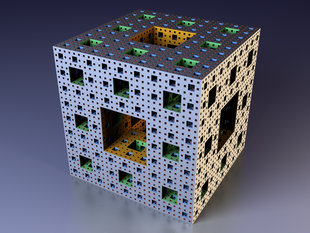Just like the Sierpinski carpet, we're removing the middle cube of each remaining cube section in each step, so here's a representation of those steps: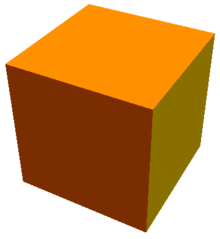Each face of the Menger Sponge is a Sierpinski carpet!

The thing that absolutely blows my mind about the Menger sponge is it has zero volume but infinite surface area! (See if you can figure out why.)

If we were to continue this process to infinity, the result would be a surface that has so many holes, that it can no longer be a surface or a solid but is instead a curve!

The Menger sponge's fractional dimension is log_3(20) because if you triple the sides of your initial cube, you get 20 copies of it.

In fact, one of my favorite math activitists, Matt Parker, and another mathematician, Laura Taalman, actually constructed a Menger sponge out of 960,000 business cards and displayed it in the UK. This is called MegaMenger and is (probably) the world's largest fractal model. Here's a view from outside:It's absolutely incredible what the math community can accomplish when they come together for projects like this! Check out Numberphile and standupmaths (Matt Parker's channel) and the other YouTube channels I have linked in the resources hub to see more of these amazing community projects, like Matt Parker's Domino Computer or Numberphile's Mile of Pi.

I'll just mention a couple more fractals related to the Sierpinski carpet and the Menger sponge here. One is the Wallis Sieve, a carpet similar to Sierpinski's, but constructed in such a way that the area of the fractal never reaches zero. Another is the Jerusalem cube, made with crosses instead of cubes. Both are worth looking into!

Finally, the Cantor set is the 1D version of the Sierpinski carpet and the Menger sponge. You can find one by cutting straight across the middle of the carpet or along one of the diagonals of the sponge. Essentially, you take a line and repeatedly remove the middle third, and you're left with a set that has measure zero (meaning the length of the set becomes zero), yet the set represents uncountable infinity because an uncountable number of elements are never deleted! (I'd absolutely love to go into more detail on this one as well. It's the idea I alluded to in an earlier post that made the mathematician Georg Cantor go crazy, so let me know in the forums/comments if you'd like more information.) Here's what it looks like:Gabriel's Horn

The last thing I'll mention is not a fractal, but it's a neat infinite shape, so I'll leave it here as a teaser. This is Gabriel's Horn, a trumpet-like solid created by rotating the graph y = 1/x over the x-axis:It turns out Gabriel's Horn (aka Gabriel's Trumpet) has a finite volume but an infinite surface area! That means if you were attempting to paint all of the inside surfaces of the horn, you would never finish the job, but you can somehow fill the entire horn with paint easily!

You do need calculus to prove this, but keep it in the back of your mind because it might be a fun thing to try to prove a couple years down the road!

Conclusion

Before I end this article, I do want to mention some other fractals that you've probably seen pop up across my website and across the internet: the Mandelbrot and Julia sets. Both of these are fractals that are incredibly complex but are somehow generated by equations that are fairly simple. You do need a bit of a background on complex numbers to understand this, but like I've said several times throughout this article, let me know if you'd like me to write about this topic as well! Here's a zoom of the Mandelbrot set that is absolutely hypnotizing to watch:

All in all, fractals make up such a rich subject in math, and what we've looked at today is just the tip of the iceberg! I hope I've been able to show you a bit of the wonder and beauty of math in all the surprising properties and connections between these mathematical objects. Before I go, I'll leave you with a couple of links and resources so you can explore fractals even more on your own:

Here's one that gives you an introduction to fractals:

And here's another that adds a bit more complexity to the subject (don't worry what I said isn't wrong; this is just even more correct):

And here's a bit more fun! Create and draw your own fractals (done here in Microsoft Paint to show you that you can do it anywhere):

I hope you enjoyed my longest and most ambitious article to date! I haven't seen a guide that quite covers everything I wanted it to, so I made my own! Leave any questions in the comments and forums and we'll discuss fractals together. :)